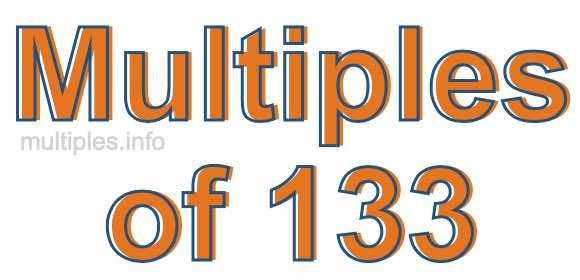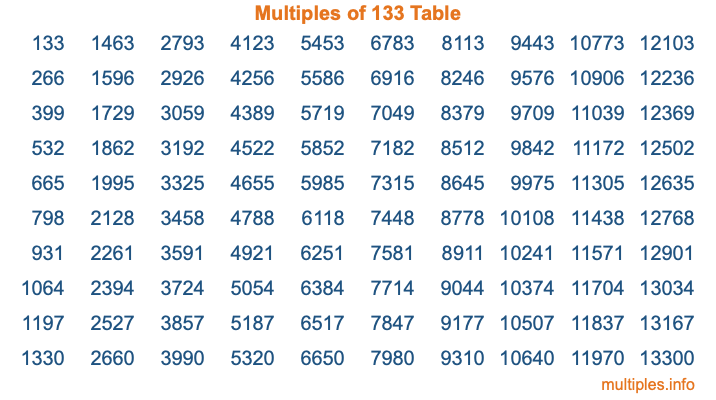Multiples of 133Welcome to the Multiples of 133 page. Here we will first teach you everything you will ever need to know about the multiples of 133, and then give you a study guide summary of everything we taught you to make sure you remember it all. Use this page to look up facts and learn information about the multiples of 133. This page will make you a multiples of one hundred thirty-three expert!

Definition of Multiples of 133
Multiples of 133 are all the numbers that when divided by 133 equal an integer. Each of the multiples of 133 are called a multiple. A multiple of 133 is created by multiplying 133 by an integer.

Therefore, to create a list of multiples of 133, you start with 1 multiplied by 133, then 2 multiplied by 133, then 3 multiplied by 133, and so on for as long as you want. Thus, the list of the first five multiples of 133 is 133, 266, 399, 532, and 665. To see a larger list of multiples of 133, see the printable image of Multiples of 133 further down on this page. We also have a category where you can choose any nth multiple of 133.

Multiples of 133 Checker
The Multiples of 133 Checker below checks to see if any number of your choice is a multiple of 133. In other words, it checks to see if there is any number (integer) that when multiplied by 133 will equal your number. To do that, we divide your number by 133. If the the quotient is an integer, then your number is a multiple of 133.

Is  a multiple of 133?

Least Common Multiple of 133 and ...
A Least Common Multiple (LCM) is the lowest multiple that two or more numbers have in common. This is also called the smallest common multiple or lowest common multiple and is useful to know when you are adding our subtracting fractions. Enter one or more numbers below (133 is already entered) to find the LCM.

Check out our LCM Calculator if you need more details about the Least Common Multiple or if you need the LCM for different numbers for adding and subtraction fractions.

nth Multiple of 133
As we stated above, 133 is the first multiple of 133, 266 is the second multiple of 133, 399 is the third multiple of 133, and so on. Enter a number below to find the nth multiple of 133.

th multiple of 133

Multiples of 133 vs Factors of 133
133 is a multiple of 133 and a factor of 133, but that is where the similarities end. All postive multiples of 133 are 133 or greater than 133. All positive factors of 133 are 133 or less than 133.

Below is the beginning list of multiples of 133 and the factors of 133 so you can compare:

Multiples of 133: 133, 266, 399, 532, 665, etc.

Factors of 133: 1, 7, 19, 133

As you can see, the multiples of 133 are all the numbers that you can divide by 133 to get a whole number. The factors of 133, on the other hand, are all the whole numbers that you can multiply by another whole number to get 133.

It's also interesting to note that if a number (x) is a factor of 133, then 133 will also be a multiple of that number (x).

Multiples of 133 vs Divisors of 133
The divisors of 133 are all the integers that 133 can be divided by evenly. Below is a list of the divisors of 133.

Divisors of 133: 1, 7, 19, 133

The interesting thing to note here is that if you take any multiple of 133 and divide it by a divisor of 133, you will see that the quotient is an integer.

Multiples of 133 Table
Below is an image of the first 100 multiples of 133 in a table. The table is in chronological order, column by column. The first column has the first ten multiples of 133, the second column has the next ten multiples of 133, and so on.The Multiples of 133 Table is also referred to as the 133 Times Table or Times Table of 133. You are welcome to print out our table for your studies.

Negative Multiples of 133
Although not often discussed or needed in math, it is worth mentioning that you can make a list of negative multiples of 133 by multiplying 133 by -1, then by -2, then by -3, and so on, to get the following list of negative multiples of 133:

-133, -266, -399, -532, -665, etc.

Multiples of 133 Summary
Below is a summary of important Multiples of 133 facts that we have discussed on this page. To retain the knowledge on this page, we recommend that you read through the summary and explain to yourself or a study partner why they hold true.

There are an infinite number of multiples of 133.

A multiple of 133 divided by 133 will equal a whole number.

133 divided by a factor of 133 equals a divisor of 133.

The nth multiple of 133 is n times 133.

The largest factor of 133 is equal to the first positive multiple of 133.

133 is a multiple of every factor of 133.

133 is a multiple of 133.

A multiple of 133 divided by a divisor of 133 equals an integer.

133 divided by a divisor of 133 equals a factor of 133.

Any integer times 133 will equal a multiple of 133.

Multiples of a Number
Here you can get the multiples of another number, all with the same attention to detail as we did for multiples of 133 on this page.

Multiples of
Multiples of 134
Did you find our page about multiples of one hundred thirty-three educational? Do you want more knowledge? Check out the multiples of the next number on our list!